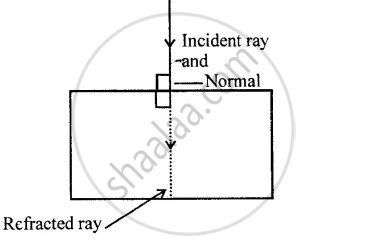ICSE Class 8CISCE
Share

# A Ray of Light Falls Normally on a Glass Slab. What is the Angle of Incidence ?V - ICSE Class 8 - Physics

ConceptRay Diagrams with Curved Mirrors Where Real Images Are Formed

#### Question

A ray of light falls normally on a glass slab. What is the angle of incidence ?

#### Solution

When a ray of light falls on a glass slab normally, angle between normal and incident ray is zero.
∴ Angle of incidence is zero.Is there an error in this question or solution?
Solution A Ray of Light Falls Normally on a Glass Slab. What is the Angle of Incidence ?V Concept: Ray Diagrams with Curved Mirrors Where Real Images Are Formed.
S